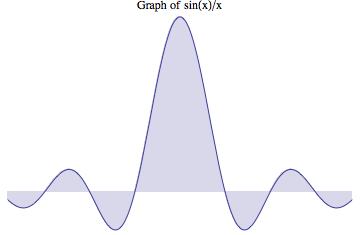# Hundredth Derivative of Sinc FunctionIn mathematics, physics, and engineering, the cardinal sine function or sinc function, denoted by $\def\sinc{\text{sinc }} \sinc x$, is defined by $\def\sinc{\text{sinc }} \sinc x=\frac{\sin x}{x}.$ The term "sinc" is a contraction of the function's full Latin name, the sinus cardinalis (cardinal sine). It was introduced by Phillip M. Woodward in his 1952 paper "Information Theory and Inverse Probability in Telecommunication" in which he said the function "occurs so often in Fourier analysis and its applications that it does seem to merit some notation of its own" and his 1953 book "Probability and Information Theory, with Applications to Radar".

Compute $m+n$, where $m$ and $n$ are relatively prime, where

$\def\sinc{\text{sinc }} \left.\frac{d^{100}}{dx^{100}}(\sinc x)\right|_{x=0}=\frac{m}{n}.$

×